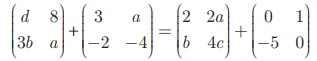Home | | Maths 10th Std | Properties of Matrix Addition and Scalar Multiplication

# Properties of Matrix Addition and Scalar Multiplication

Let A, B, C be m ×n matrices and p and q be two non-zero scalars (numbers). Then we have the following properties.

Properties of Matrix Addition and Scalar Multiplication

Let A, B, C be m ×n matrices and p and q be two non-zero scalars (numbers). Then we have the following properties.

(i) A + B = B + A [Commutative property of matrix addition]

(ii) A + (B + C) = (A + B) +C [Associative property of matrix addition]

(iii) ( pq)A = p(qA) [Associative property of scalar multiplication]

(iv) IA=A [Scalar Identity property where I is the unit matrix]

(v) p(A + B) = pA + pB [Distributive property of scalar and two matrices]

(vi) ( p + q )A = pA +qA [Distributive property of two scalars with a matrix]

The null matrix or zero matrix is the identity for matrix addition.

Let A be any matrix.

Then, A + O = O + A = A where O is the null matrix or zero matrix of same order as that of A

If A be any given matrix then –A is the additive inverse of A.

In fact we have A + (−A) = (−A) + A = O

### Example 3.62

Find the value of a, b, c, d, x, y from the following matrix equation.### Solution

First, we add the two matrices on both left, right hand sides to getEquating the corresponding elements of the two matrices, we have

d + 3 = 2  gives d = –1

8 + a = 2a + 1 gives a = 7

3b − 2 = b – 5 gives b = -3/4

Substituting a = 7 in a − 4 = 4c gives c = 3/4

Therefore, a = 7, b = − 3/2, c = 3/4, d = –1.

Example 3.63

Ifcompute the following : (i) 3A + 2B – C (ii) 1/2 A -3/2 B

SolutionStudy Material, Lecturing Notes, Assignment, Reference, Wiki description explanation, brief detail
10th Mathematics : UNIT 3 : Algebra : Properties of Matrix Addition and Scalar Multiplication |